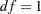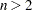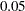Modification Indices

While fitting structural equation models is mostly a confirmatory analytic procedure, it does not prevent you from exploring what might have been a better model given the data. After fitting your theoretical structural equation model, you might want to modify the original model in order to do one of the following:

• add free parameters to improve the model fit significantly

• reduce the number of parameters without affecting the model fit too much

The first kind of model modification can be achieved by using the Lagrange multiplier (LM) test indices. Parameters that have the largest LM indices would increase the model fit the most. In general, adding more parameters to your model improves the overall model fit, as measured by those absolute or standalone fit indices (see the section Overall Model Fit Indices for more details). However, adding parameters liberally makes your model more prone to sampling errors. It also makes your model more complex and less interpretable in most cases. A disciplined use of LM test indices is highly recommended. In addition to the model fit improvement indicated by the LM test indices, you should also consider the theoretical significance when adding particular parameters. See Example 26.27 for an illustration of the use of LM test indices for improving model fit.

The second kind of model modification can be achieved by using the Wald statistics. Parameters that are not significant in your model may be removed from the model without affecting the model fit too much. In general, removing parameters from your model decreases the model fit, as measured by those absolute or standalone fit indices (see the section Overall Model Fit Indices for more details). However, for just a little sacrifice in model fit, removing non-significant parameters increases the simplicity and precision of your model, which is the virtue that any modeler should look for.

Whether adding parameters by using the LM test indices or removing unnecessary parameters by the Wald statistics, you should not treat your modified model as if it were your original hypothesized model. That is, you should not publish your modified model as if it were hypothesized a priori. It is perfectly fine to use modification indices to gain additional insights for future research. But if you want to publish your modified model together with your original model, you should report the modification process that leads to your modified model. Theoretical justifications of the modified model should be supplemented if you want to make strong statements to support your modified model. Whenever possible, the best practice is to show reasonable model fit of the modified model with new data.

To modify your model either by LM test indices or Wald statistics, you can use the MODIFICATION or MOD option in the PROC CALIS statement. To customize the LM tests by setting specific regions of parameters, you can use the LMTESTS statements. PROC CALIS computes and displays the following default set of modification indices:

• univariate Lagrange multiplier (LM) test indices for parameters in the model
These are second-order approximations of the decrease in thevalue that would result from allowing the fixed parameter values in the model to be freed to estimate. LM test indices are ranked within their own parameter regions in the model. The ones that suggest greatest model improvements (that is, greatestdrop) are ranked first. Depending on the type of your model, the set of possible parameter regions varies. For example, in a RAM model, modification indices are ranked in three different parameter regions for the covariance structures: path coefficients, variances of and covariances among exogenous variables, and the error variances and covariances. In addition to the value of the Lagrange multiplier, the corresponding-value () and the approximate change of the parameter value are displayed.

If you use the LMMAT option in the LMTESTS statement, LM test indices are shown as elements in model matrices. Not all elements in a particular model matrix will have LM test indices. Elements that are already free parameters in the model do not have LM test indices. Instead, the parameter names are shown. Elements that are model restricted values (for example, direct path from a variable to itself must be zero) are labeled Excluded in the matrix output. When you customize your own regions of LM tests, some elements might also be excluded from a custom set of LM tests. These elements are also labeled as Excluded in the matrix output. If an LM test for freeing a parameter would result in a singular information matrix, the corresponding element in the matrix is labeled as Singular.

• univariate Lagrange multiplier test indices for releasing equality constraints
These are second-order approximations of the decrease in thevalue that would result from the release of equality constraints. Multiple equality constraints containingparameters are tested successively insteps, each assuming the release of one of the equality-constrained parameters. The expected change of the parameter values of the separated parameter and the remaining parameter cluster are displayed, too.

• univariate Lagrange multiplier test indices for releasing active boundary constraints
These are second-order approximations of the decrease in thevalue that would result from the release of the active boundary constraints specified in the BOUNDS statement.

• stepwise multivariate Wald statistics for constraining free parameters to 0
These are second-order approximations of the increases invalue that would result from constraining free parameters to zero in a stepwise fashion. In each step, the parameter that would lead to the smallest increase in the multivariatevalue is set to 0. Besides the multivariatevalue and its-value, the univariate increments are also displayed. The process stops when the univariate-value is smaller than the specified value in the SLMW= option, of which the default value is.

All of the preceding tests are approximations. You can often obtain more accurate tests by actually fitting different models and computing likelihood ratio tests. For more details about the Wald and the Lagrange multiplier test, refer to MacCallum (1986), Buse (1982), Bentler (1986), or Lee (1985). Note that relying solely on the LM tests to modify your model can lead to unreliable models that capitalize purely on sampling errors. See MacCallum, Roznowski, and Necowitz (1992) for the use of LM tests.

For large model matrices, the computation time for the default modification indices can considerably exceed the time needed for the minimization process.

The modification indices are not computed for unweighted least squares or diagonally weighted least squares estimation.